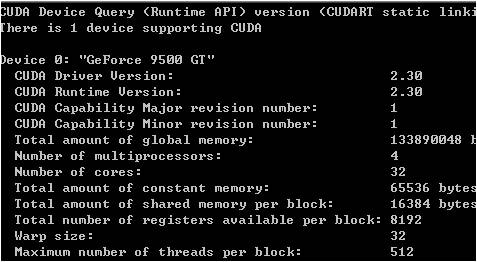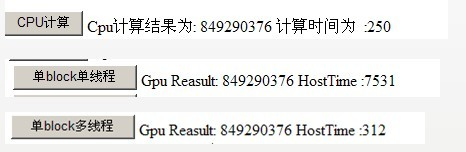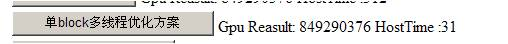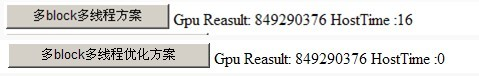# CUDA并行计算框架

2015-9-9 12:25| 发布者: 炼数成金_小数| 查看: 1847| 评论: 0|原作者: 熬夜的虫子|来自: cnblogs存储GPU内核CUDA并行计算函数 从这部分开始 结合虫子的demo程序给大家分析下cuda的性能与可行性。一、先概述下实现流程。CUDA在执行的时候是让host里面的一个一个的kernel按照线程网格（Grid）的概念在显卡硬件（GPU）上执行。每一个线程网格又可以包含多个线程块（block），每一个线程块中又可以包含多个线程（thread）。每一个kernel交给每一个Grid来完成。当要执行这些任务的时候，每一个Grid又把任务分成一部分一部分的block，block再分线程来完成。每个Grid中的任务是一定的。二维线程块的索引关系为如下：unsigned int xIndex = blockDim.x * blockIdx.x + threadIdx.x; unsigned int yIndex = blockDim.y * blockIdx.y + threadIdx.y;block中的每个线程都有自己的寄存器和local memory，block中的所有线程共享一个shared memory，一个grid共享一个global memory。每一个时钟周期内，warp（一个block里面一起运行的thread）包含的thread数量是有限的，现在的规定是32个。一个block中含有16个warp。所以一个block中最多含有512个线程每次Device（就是显卡）只处理一个grid。下面说明一下硬件的执行模型。     假如出于某种原因，公司的办公室被征用了搞活动。只留下一个小房间来给开发团队。每一个时钟周期内按照wrap（就理解为运行的时候，一个block里面一起运行的thread，例如block里面有512个thread，但是每次只有32个thread在运行，那么这32个thread就是一个运行的warp组 线程束）。每一个warp里面包含的thread数量是有限的，现在的规定是32个。将来不知道会不会有变化，这个只有CUDA开发人员知道了。每次Device（就是显卡）只处理一个grid(在未来支持directX11 的硬件中这一限制可能被解除)。假如我们一个部门有x个人，办公室内有N个桌子，每张桌子可以坐32个人。然后轮流来开发….。这里的桌子可以理解成multiprocessor（多处理器）。每个sm中包含8个标量流处理器（sp）。GPU所谓的多核中核的概念就是sp的数量。Cuda中的kernel函数实质上是以block为单位执行的。同一block中需要共享数据，因此他们必须在同一个SM中发射，而block中的每一个线程则被发射到sp上去执行。疑点：既然有这样的线程簇限制、为何还要设置高于warp线程数的线程。二、demo 安装部署方面driver、toolkit、sdk顺序安装好。Cuda的项目支持4种调试方式release、debug、emurelease、emudubug。前2个是需要gpu真正的支持cuda后者是cpu模拟gpu。至于你的电脑能否支持cuda 可以运行下deviceQuery.exe程序图中我们关注一下几点就可以了，首先 有一个支持cuda的设备。计算能力1，局存储器的大小，核的数量，多处理器的数量，常量存储器的大小、每个block的共享存储器的大小、wrap的线程数等等。想看cuda在图形领域的应用可以运行这个smokeParticles.exe程序哦。在我的demo中，cpp文件主要是处理一些cpu端的处理、cu文件通常是与gpu核函数和cuda api的一些内容。其中My_kernel封装了具体的核函数实现方法。Cudatool项目就是cuda的应用程序，CudaProviders是我连接C#与cuda之间的驱动、CudaWeb就是我们平常的web项目。CUDAWinApp这个就是一些小的功能演示。下面介绍下cuda的函数类型限定符。__device__ 在设备上执行、只能在设备上调用。__global__ 用于声明内核函数、在设备上执行只能从主机端调用。__host__ 在主机端执行，只能从主机端调用，默认。__device__与__global__不支持递归，函数体内不能声明静态变量、参数数目不可变化，不能对device取指针。__global__与__host__不能连用。__global__只能返回空，调用__global__函数必须声明其执行配置、__global__函数的调用是异步的、__global__参数的值目前是通过共享存储器传递，总的大小不能超过256byte。变量类型限定符分为__device__（变量存在设备端上）、__constant__（存在常数存储器空间）、__share__（block的共享存储器）、volatile关键字 当线程间数据可能互相影响变换时使用。bool InitCUDA(void){    int count = 0;    int i = 0;    cudaGetDeviceCount(&count);    if(count == 0) {        fprintf(stderr, "没显卡.\n");        return false;    }    for(i = 0; i < count; i++) {        cudaDeviceProp prop;        if(cudaGetDeviceProperties(&prop, i) == cudaSuccess) {            if(prop.major >= 1) {                break;            }        }    }    if(i == count) {        fprintf(stderr, "没支持CUDA的显卡.\n");        return false;    }    cudaSetDevice(i);    printf("初始化ok.\n");    return true;}这个方法里面最重要是cudaGetDeviceCount和cudaGetDeviceProperties函数，这个cuda开发库的自带函数。通过这个函数我们可以判断出可用于执行的计算能力大于1.0的设备数量。 cudaMalloc((void**) &gpudata, sizeof(int) * DATA_SIZE); 为输入数据分配显存空间。cudaMemcpy(gpudata, data, sizeof(int) * DATA_SIZE,        cudaMemcpyHostToDevice);将内存中的数据拷贝到显存中去。这里cudaMemcpyHostToDevice从内存拷贝到显存，cudaMemcpyDeviceToHost从显存拷贝到内存，cudaMemcpyDeviceToDevice将全局存储器上的数据拷贝到同一cuda上下文的全局存储器的另一区域中去。cudaMalloc((void**) &result, sizeof(int) * BLOCK_NUM);为输出数据分配显存空间。switch(mode)        {        case 1:            sum<<<1, 1, 0>>>(gpudata, result);            break;        case 3://线程            sum_Thread<<<1, THREAD_NUM, 0>>>(gpudata, result);            break;        case 4://            sum_ThreadOptimization<<<1, THREAD_NUM, 0>>>(gpudata, result);            break;        case 5://             sum_Block<<>>(gpudata, result);            break;        case 6://            sum_Block_sync<<>>(gpudata, result);            break;        }内核函数。其中<<<>>>运算符对kernel函数完整的执行参数配置形式是<<>>.DG用于定义整个grid的维度和尺寸。Dim3类型(cuda的内置类型在定义类型为 dim3 的变量时，未指定的任何组件都将初始化为 1。)。上面的形式准确来写应该是这样。Dim3 grid（num_blocks,1,1）Dim3 threads（num_threads,1,1）Sum<<>>dim3 DG(Dg.x,Dg.y,1)中每行有DG.x个block，实际上只有前2个不为1，每列Dg.y个block。第三维恒定为1。Db为dim3类型，用于定义每个block的维度和尺寸。Dim3 Db（Db.x,Db.y,Db.z）中每行有Db.x个线程，每列有Db.y个线程，高度为db.z。参数ns是一个可选参数，用于设置每个block除了静态分配的shared memory以外，最多能够分配的shared memory大小，参数s是一个cudastream_t类型的可选参数，默认为0。#define DATA_SIZE 1048576*15#define THREAD_NUM   256#define BLOCK_NUM   32__global__ static void sum(int *num, int* result){    int sum = 0;    int i;    for(i = 0; i < DATA_SIZE; i++) {        sum += num[i] + num[i] + num[i] + num[i] + num[i] + num[i]+ num[i] + num[i] + num[i] + num[i] + num[i] + num[i];    }    *result = sum;}这个内核函数很简单，因为是单线程，将显存中的数据每个元素加10遍返回结果。__global__ static void sum_Thread(int *num, int* result){    const int tid = threadIdx.x;    const int size = DATA_SIZE / THREAD_NUM;           int sum = 0;    int i;        for(i = tid * size; i < (tid + 1) * size; i++) {       sum += num[i] + num[i] + num[i] + num[i] + num[i] + num[i]+ num[i] + num[i] + num[i] + num[i] + num[i] + num[i];    }    result[tid] = sum;        }这个是个单block多线程的内核函数，大家看下里面的区别。按照线程数量，每个线程处理对应地址的数据，最后汇总，这里每个线程所分配的资源都是线性的.下面我们来比较下目前这3个方法的准确性已经性能差异。在 CUDA 中，一般的数据复制到的显卡内存的部份，称为 global memory。这些内存是没有 cache 的，而且，存取 global memory 所需要的时间是非常长的，通常是数百个 cycles。由于我们的程序只有一个 thread，所以每次它读取 global memory 的内容，就要等到实际读取到数据、累加到 sum 之后，才能进行下一步。这就是为什么它的表现会这么的差。权权上次也说了 如果cpu来用多线程来做效率会怎么样。这边做下说明，在低数量级的运算中 cpu确实会比gpu高的，应该按照综合性能来说cpu还是要比gpu强。但是对于高数量级的运算，根据cpu和gpu的结构来看，2者的差异还是相当大的。而且对于在gpu并行计算的规则和方法 cuda是提供一套成品的框架，如果用cpu的话，期待微软在.net 4.0中提出的并行计算的概念吧。下面我们继续深入如何在并行计算中优化自己的方案。就拿上面的单block多线程来说，有大量的 threads 在同时执行，那么当一个 thread 读取内存，开始等待结果的时候，GPU 就可以立刻切换到下一个 thread，并读取下一个内存位置。因此，理想上当 thread 的数目够多的时候，就可以完全把 global memory 的巨大 latency 隐藏起来了。前面的程序，虽然看起来是连续存取内存位置（每个 thread 对一块连续的数字计算），但是我们要考虑到实际上 thread 的执行方式。前面提过，当一个 thread 在等待内存的数据时，GPU 会切换到下一个 thread。也就是说，实际上执行的顺序是类似thread 0 -> thread 1 -> thread 2 -> ...所以我们应该这样设计，让 thread 0 读取第一个数字，thread 1 读取第二个数字…依此类推。下面就是我们的第一个优化方案。__global__ static void sum_ThreadOptimization(int *num, int* result){    const int tid = threadIdx.x;    int sum = 0;    int i;      for(i = tid; i < DATA_SIZE; i += THREAD_NUM) {       sum += num[i] + num[i] + num[i] + num[i] + num[i] + num[i]+ num[i] + num[i] + num[i] + num[i] + num[i] + num[i];    }    result[tid] = sum;        }然后我们看下效率然后我们看下gpu更强大的运算能力，多block运算。__global__ static void sum_Block(int *num, int* result    ){    const int tid = threadIdx.x;    const int bid = blockIdx.x;    int sum = 0;    int i;    for(i = bid * THREAD_NUM + tid; i < DATA_SIZE;        i += BLOCK_NUM * THREAD_NUM) {       sum += num[i] + num[i] + num[i] + num[i] + num[i] + num[i]+ num[i] + num[i] + num[i] + num[i] + num[i] + num[i];    }    result[bid * THREAD_NUM + tid] = sum;   }其实就是算法上由一维变成了二维。但是在回拷内存的时候差异要比较一下。这个是单线程的。if(mode == 1)        {            int sum3;            cudaMemcpy(&sum3, result, sizeof(int), cudaMemcpyDeviceToHost);            sprintf(s2,"%d",sum3);                }这个是多线程的。else if(mode<5)        {            int sum[THREAD_NUM];             cudaMemcpy(&sum, result, sizeof(int) * THREAD_NUM,                cudaMemcpyDeviceToHost);            int final_sum = 0;            for(int i = 0; i < THREAD_NUM; i++) {                final_sum += sum[i];             }            sprintf(s2,"%d",final_sum);                   }这个是多block的。else if(mode==5)        {            int sum_block[THREAD_NUM * BLOCK_NUM];            cudaMemcpy(&sum_block, result, sizeof(int) * THREAD_NUM * BLOCK_NUM,                cudaMemcpyDeviceToHost);            int  final_sum = 0;            for(int i = 0; i < THREAD_NUM * BLOCK_NUM; i++) {                final_sum += sum_block[i];            }            sprintf(s2,"%d",final_sum);        }下面我们重点看下优化方案。前面提过，一个 block 内的 thread 可以有共享的内存，也可以进行同步。我们可以利用这一点，让每个 block 内的所有 thread 把自己计算的结果加总起来。__global__  void sum_Block_sync(int* num, int* result){    extern __shared__ int shared[];    const int tid = threadIdx.x;    const int bid = blockIdx.x;    int i;      shared[tid] = 0;    for(i = bid * THREAD_NUM + tid; i < DATA_SIZE;        i += BLOCK_NUM * THREAD_NUM) {       shared[tid] += num[i] + num[i] + num[i] + num[i] + num[i] + num[i]+ num[i] + num[i] + num[i] + num[i] + num[i] + num[i];    }    __syncthreads();    if(tid == 0) {        for(i = 1; i < THREAD_NUM; i++) {            shared += shared[i];        }        result[bid] = shared;    }   }利用 __shared__ 声明的变量表示这是 shared memory，是一个 block 中每个 thread 都共享的内存。它会使用在 GPU 上的内存，所以存取的速度相当快，不需要担心 latency 的问题。__syncthreads() 是一个 CUDA 的内部函数，表示 block 中所有的 thread 都要同步到这个点，才能继续执行。--- ---！已经相当牛了。这样的话 在cpu回拷那块只需要block数目的数据就可以了。else if(mode == 6)        {            int sum_sync[BLOCK_NUM];            cudaMemcpy(&sum_sync, result, sizeof(int) * BLOCK_NUM,                cudaMemcpyDeviceToHost);            int final_sum = 0;            for(int i = 0; i < BLOCK_NUM; i++) {                final_sum += sum_sync[i];            }             sprintf(s2,"%d",final_sum);        }

• 大数据
• 商业智能
• 量化投资
• 科学探索
• 创业

## 热门文章GMT+8, 2020-10-30 12:42 , Processed in 0.160736 second(s), 23 queries .